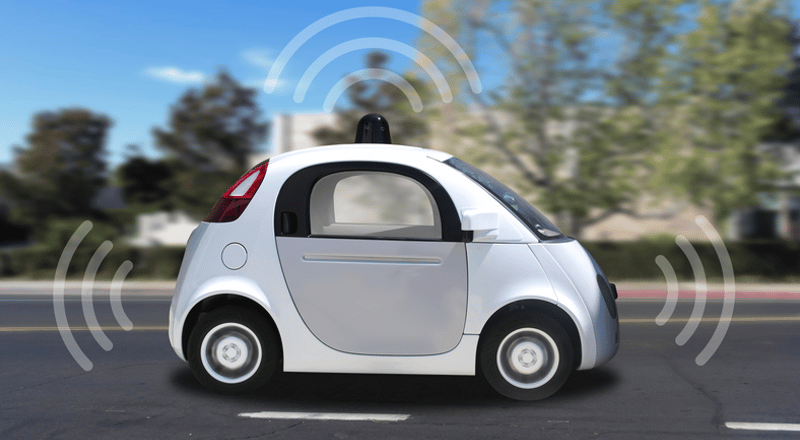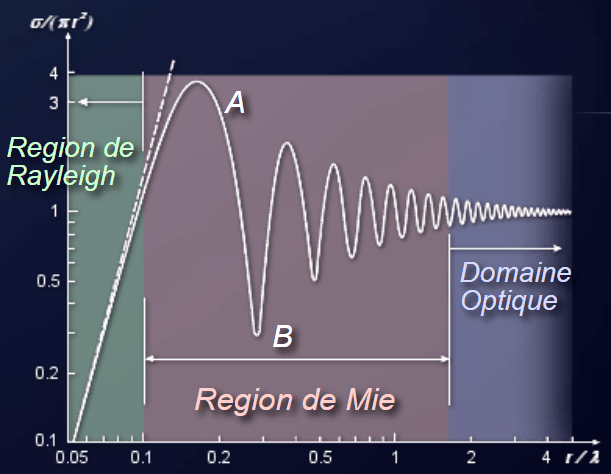# The Sandbox: the AVSandbox knowledge hub

The Sandbox knowledge hub discusses many of the crucial issues affecting the development, engineering, use and regulation of Autonomous Vehicles.Scroll to explore## Ray Tracing Algorithms for Radar Modeling

In previous blog posts we have described various aspects of the radar simulator such as the antenna (Modeling of Phased Array Antenna), the interaction with matter (Radar Modeling – Reflection of an EM wave) and the validation process (Verification & Validation of a Simulation Model). We will now talk about the algorithm it is using: ray tracing. This description is aimed at those developing their own ray tracing models, or others who want an overview of how it works and want to follow the future development of our radar model.

### Transmission

The simplest model for propagation is the Friis equation between 2 antennas of matching impedance and polarization in free space.This accounts for direct transmission between transmitter and receiver, but when objects are in the way, we must account for reflection, diffraction and scattering. Reflection and scattering are covered in our previous blog : Radar Modeling – Reflection of an EM wave. We will first describe the different models of EM waves and where ray tracing fits within them. And then we will briefly talk about diffraction.

### Model Overview

There are 3 types of models :

• Theoretical models : Usually fast and accurate, but cannot be too complex.
• Empirical models : Depend on the given environment the model is based on, and inappropriate for MIMO (not able to provide accurate space-time or angle-delay results).
• Computational models (CEM) : Amongst CEM, we can quote :
• Ray Tracing (RT) : Numerical method solving Maxwell’s equations
• Full wave method : Very costly when the target is large in regard to the wavelength :
• Finite Element Method (FEM)
• Finite-Difference Time Domain (FDTD)
• Method of Moment (MoM)

There are 2 calculation methods :

• Rigorous method : very precise but not very effective for problems past a few wavelengths.
• Asymptotic method : or high frequency approximation in the sense that we use a simplified version of the Maxwell’s equation for high frequencies, and the model gets more accurate as the frequency increases. Amongst asymptotic methods we can rely on:
• Field Theory :
• Geometrical Optics (GO)
• Uniform Theory of Diffraction (UTD)
• Other models of diffraction
• Current Theory :
• Physical Optics (PO)
• Incremental Theory of Diffraction (ITD)

In this document we will only deal with ray tracing. The other approaches, FEM, FDTD and MoM, are slow because they rely on the discretisation of differential equations. Equally, we are only interested in the Asymptotic methods because of the large scene. However, different models can be used to simulate the scene in an optimised way. GO will be used to determine the direction of the rays, and UTD will be used at the interface to calculate the direction and module of the reflected rays.

### Shooting and Bouncing Rays

Once the Ray tracing algorithm has been selected, there are different ways to implement it. In an urban environment, you cannot only consider the rays from a source location to a target, but have to take into account all the reflections, diffractions and other “paths” rays can take to travel. This is called multipath, that is complex to model and requires fast computation and precise calculation of the fields. For that reason, the Shooting and Bouncing Rays (SBR) method is commonly used. This method is made of three major steps :

• Ray launching : All your rays emanate from the same source (the antenna), and are launched onto the scene. The beam pattern of your antenna will determine the power of each ray in each direction, it is therefore important to keep in mind eventual blind spots and to try and uniformly distribute the rays across the scene.
• Ray tracing : All your rays are then propagating throughout the scene and you keep track of all their information (power, polarisation, direction, ..). Every time a ray intersects any object, you have to work out the resulting rays (from reflection, diffraction, ..).
• Ray reception : You only stop when your ray comes back to your antenna (or when its power isn’t enough to keep it going). There, you calculate the object seen by the radar based on the time and direction of the returning ray. The same object may be seen several times in several places because of the multipath effect. These duplicates of the object are called ghost targets and need to be dealt with in a signal processing step.

### High Frequency approximation

Ray Tracing relies on an approximation of Maxwell’s equations to reduce memory needs and simulation time. Let’s go through this high frequency approximation. On the image below, the normalized backscattering cross-section (RCS) of a sphere is calculated (vertical axis) in different frequency domains (horizontal axis). Three regions appear :• the Rayleigh region (green), where the sphere is much smaller than the wavelength. In this region the energy diffused by the sphere will be directly proportional to the size of the sphere. It is useful to determine rainfall rates for example.
• the Mie region (pink), where the sphere is of similar size as the wavelength. In that case, a small variation in the size of the sphere observed will give you massive changes in your observations.
• the Optical domain (or far field, purple), where the sphere is big compared to the wavelength.

Thanks to the frequencies used by automotive radar that tend to increase (moving from 24 GHz to 77 GHz), we can place ourselves in the optical region and therefore avoid the resonance issues of the Mie region. In this region, the RCS becomes independent of frequency, thus allowing for a simplification of Maxwell’s equations. This approximation gets better as frequency increases.

### Diffraction

Diffraction is described by Huygens–Fresnel principle. It is treated separately from reflection and scattering because it usually cannot be described by Geometrical Optics (it is a wave effect, whereas GO describes lights as a ray). Therefore a ray tracing algorithm must include at least 2 types of modeling to account for all those phenomenon. Diffraction is described by Huygens–Fresnel principle. It is treated separately from reflection and scattering because it usually cannot be described by Geometrical Optics (it is a wave effect, whereas GO describes lights as a ray). Therefore a ray tracing algorithm must include at least 2 types of modelling to account for all those phenomenon. Diffraction has been an intense object of study since the 1880’s (Rayleigh and Kirchhoff). The early calculations focused on easy shapes but most of the research since the 40’s aimed to obtain formulas for the calculation of diffractions due to knife edges. As per section “Model Overview”, diffraction can be modelled using field theory or current theory. In our algorithm we chose Uniform Theory of Diffraction. It is using field theory and also high frequency approximation. It approximates the near-field with a diffraction factor for each ray that is diffracted. We can then decide how many of those we actually model. It is not straightforward to implement because you need to detect sharp edges and therefore need a “volume” around your ray to detect an abrupt change in the object you are hitting.

### Conclusion

We could only give you a brief overview of the techniques and algorithms used for radar modeling, each having pros and cons. We could only give you a brief overview of the techniques and algorithms used for radar modelling, each having pros and cons. In our opinion, ray tracing is the best way to model an automotive radar because it is precise enough to give exploitable results and complete enough to account for most physical phenomenon. It scales well with the size of a scene surrounding a car, and uses the relatively high frequency of modern radars to increase calculation speed and reduce memory needs.

Written by: Geoffroy Heurtier – Project Engineer

Please get in touch if you have any questions or have got a topic in mind that you would like us to write about. You can submit your questions / topics via: Tech Blog Questions / Topic Suggestion

### The AVSandbox Knowledge Hub

Discover more about what makes AVSandbox unique. Explore our AVSandbox knowledge hub and find out about the issues, challenges and exciting developments that are behind the growth of the market for autonomous vehicles and advanced driver assistance systems.

### Tackling High Development Costs: How AVSandbox Can Accelerate Your Autonomous Vehicle Deployment

Reducing costs of autonomous vehicle development without compromising AV Safety The development and successful deployment of autonomous vehicles is ...

### Determinist Traffic Simulation

Introduction In my previous blog deterministic scenario simulation, I detailed why we define our simulator deterministic and what is ...

### Deterministic Scenario Simulation

Introduction As initially introduced by the Providing the Safety Case: is Deterministic or Stochastic?, a stochastic simulation containing several ...

Go to Top